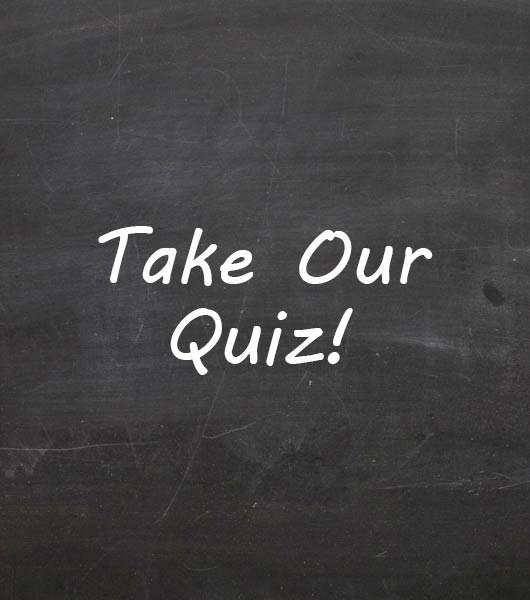Intro To Chapter 2 Quiz

14 Questions | Total Attempts: 36Settings• 1.
11BHow many protons?
• A.

4

• B.

5

• C.

6

• D.

10

• E.

11

• 2.
11BHow many electrons?
• A.

4

• B.

5

• C.

6

• D.

10

• E.

11

• 3.
11BHow many neutrons?
• A.

4

• B.

5

• C.

6

• D.

10

• E.

11

• 4.
18O-2How many protons?
• A.

7

• B.

8

• C.

9

• D.

10

• E.

18

• 5.
18O-2How many electrons?
• A.

7

• B.

8

• C.

9

• D.

10

• E.

18

• 6.
18O-2How many neutrons?
• A.

7

• B.

8

• C.

9

• D.

10

• E.

18

• 7.
If an element has 11 protons, 12 neutrons and 10 electrons, what is the element?
• A.

Neon

• B.

Sodium

• C.

Magnesium

• D.

Carbon

• E.

Oxygen

• 8.
If an element has 11 protons, 12 neutrons and 10 electrons, what is its atomic mass?
• A.

20

• B.

21

• C.

22

• D.

23

• E.

24

• 9.
If an element has 11 protons, 12 neutrons and 10 electrons, what is its atomic number?
• A.

10

• B.

11

• C.

12

• D.

21

• E.

23

• 10.
If an element has 11 protons, 12 neutrons and 10 electrons, what is its net charge?
• A.

-2

• B.

-1

• C.

0

• D.

+1

• E.

+2

• 11.
18O    and       16OThese two atoms are...
• A.

Ions

• B.

• C.

Isotopes

• D.

Acids

• E.

Neutrons

• 12.
42Ca+2For this atom, how many electrons?
• A.

18

• B.

19

• C.

20

• D.

21

• E.

22

• 13.
42Ca+2For this atom, how many neutrons?
• A.

18

• B.

19

• C.

20

• D.

21

• E.

22

• 14.
42Ca+2For this atom, if you were to add one proton, which of the features of the atom would change?
• A.

42

• B.

Ca

• C.

+2

• D.

Ca and 42

• E.

Ca, 42, and +2Back to top